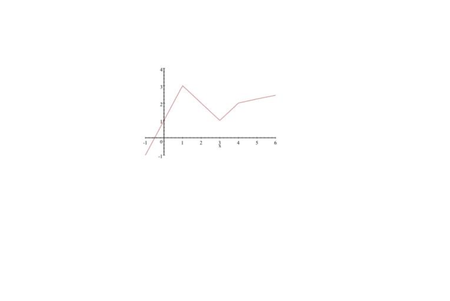# Find: Use the graph of y=g'(x) , below, to estimate point(s) for the function g(x) as well as...

## Question:

Use the graph of {eq}y=g'(x) {/eq} , below, to estimate critical point(s) for the function {eq}g(x) {/eq} as well as the intervals on which {eq}g(x) {/eq} is concave up and concave down. Explain your reasoning.## Finding Critical Points and Concavity of Function From Graph

The graph of a function is given and we use the illustration to estimate critical points for the function. Critical points are points on the domain of the function which may be relative minima or maxima or neither. The second derivative test is used to determine whether a critical point is a relative maxima or minima when the algebraic form of the function is given. This is followed by locating points of inflection of the function which are points where the second derivative of function is zero and are points where the concavity of the function changes (from concave up to concave down OR from concave down to concave up).

## Answer and Explanation:

From the definition, the critical points for a function {eq}y=g(x) {/eq} are points {eq}x {/eq} in its domain for which either {eq}g'(x) = 0 {/eq} or {eq}g'(x) {/eq} does not exist.

From the graph given us:

(i) {eq}g'(-0.5)=0 {/eq} since the graph of {eq}g'(x) {/eq} crosses the x-axis at {eq}x=-0.5. {/eq}

(ii) There are no points {eq}x {/eq} for which {eq}g'(x) {/eq} is not defined since the graph of {eq}g'(x) {/eq} is continuous on its domain.

Hence the only critical point for {eq}g(x) {/eq} is {eq}x=-0.5. {/eq}

To find points of inflection that lead to intervals of concavity, we need to solve the equation {eq}x {/eq} for which {eq}g''(x)=0. {/eq}

But note from the slopes of the straight lines in the graph of {eq}g'(x) {/eq} that we have the following:

{eq}\displaystyle g''(x) = \left\{ \begin{array}{l} 2 \quad x \in (-1,1) \\ -1 \quad x \in (1,3) \\ 1 \quad x \in (3,4) \\ 0.25 \quad x \in (4,6) \end{array} \right. {/eq}

Hence the equation {eq}g''(x)=0 {/eq} has no solution. Therefore, the function {eq}g(x) {/eq} has no points of inflection and intervals of concavity do not exist.

#### Learn more about this topic:Finding Critical Points in Calculus: Function & Graph

from CAHSEE Math Exam: Tutoring Solution

Chapter 8 / Lesson 9
163K